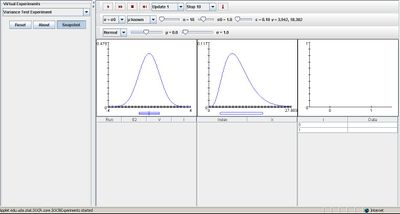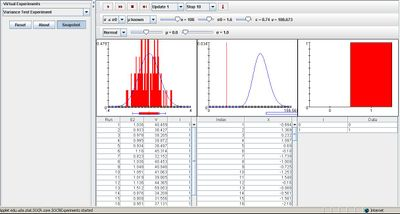# SOCR EduMaterials Activities VarianceTestExperiment

## Description

The experiment is to select a random sample of size n from a selected distribution and then test a hypothesis about the standard deviation d at a specified significance level. The distribution can be selected from a list box; the options are the N(μ,σ2), Γ, and uniform distributions. In each case, the appropriate parameters and the sample size n can be varied with scroll bars. The significance level can be selected with a list box, as can the type of test: two-sided, left-sided, or right-sided. The boundary point d0 between the null and alternative hypotheses can be varied with a scroll bar. The density of the distribution, as well as m and d, are shown in blue in the first graph; d0 is shown in green. The test can be constructed under the assumption that the distribution mean is known or unknown. In the first case, the test statistic has the χ2 distribution with n degrees of freedom; in the second case the test statistics has the χ2 distribution with n - 1 degrees of freedom. The density and the critical values of the test statistic V are shown in the second graph in blue. On each update, the sample density is shown in red in the first graph and the sample values are recorded in the first table. The sample standard deviation S is shown in red in the first graph and the value of the test statistic V is shown in red in the second graph. The variable I indicates the event that the null hypothesis is rejected. On each update, S2, V, and I are recorded in the second table. Note that the null hypothesis is reject (I = 1) if and only if the test statistic V falls outside of the critical values. Finally, the empirical density of I is shown in red in the last graph and recorded in the last table.

## Goal

The Variance Test Experiment models the hypothesis testing experiment for the variance in the standard normal model. It should help others develop a better understanding of hypothesis testing and allow them to develop a better generalization of variance hypothesis testing experiments.

## Experiment

Go to the SOCR Experiment [SOCR Experiment] and select the Variance Test Experiment from the drop-down list of experiments on the top left. The image below shows the initial view of this experiment:When pressing the play button, one trial will be executed and recorded in the distribution table below. The fast forward button symbolizes the nth number of trials to be executed each time. The stop button ceases any activity and is helpful when the experimenter chooses “continuous,” indicating an infinite number of events. The fourth button will reset the entire experiment, deleting all previous information and data collected. The “update” scroll indicates nth number of trials (1, 10, 100, or 1000) performed when selecting the fast forward button and the “stop” scroll indicates the maximum number of trials in the experiment.

For any distribution, varying parameter n changes the shape of the distribution graph on the right. Modifying the null hypothesis affects the bar under the first distribution graph and changing alpha affects the bar under the second distribution graph. Adjusting the specific parameters for the selected distribution also creates an impact on the overall result of the experiment.

Notice that the larger the values are for the null standard deviation, alpha, or both, the larger the proportion is for value 1 in the last displayed image on the right. The figure below demonstrates this:## Applications

The Variance Test Experiment is a simple application that demonstrates hypothesis testing under different distribution situations. It may be used in many different activities such as:

Suppose rolling a fair die gives a consistent population outcome of six. Let the sample size be 100 rolls of the die. If the distribution is normal, using this applet can help illustrate the outcome of the experiment.

Suppose we want to test a variance gamma option pricing model between American and European style. By using this applet, conclusions may be drawn as the two options are compared and observed to approximate which market prices are better.